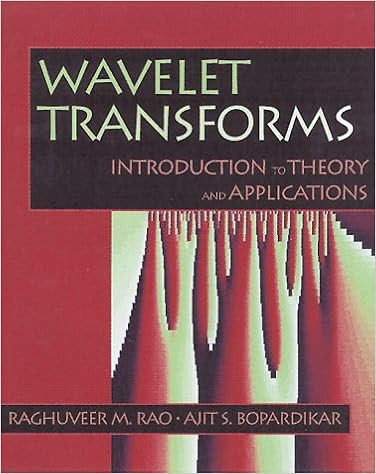## Thursday, July 30, 2015

### Wavelet Transforms: Introduction to Theory & Applications by Raghuveer M. Rao, Ajit S. Bopardikar*Written by researchers Raghuveer M. Rao and Ajit S. Bopardikar, Wavelet Transforms provides engineers, scientists, and students with a practical understanding of wavelet transforms and their properties. The authors introduce the underlying theory of the transform by presenting a wide range of applications, such as signal processing, image processing, and communications. *This book identifies problems for which wavelet transform techniques are well-suited, shows how to implement wavelet transforms efficiently, and explains how to choose or design appropriate wavelets for a given application. In Chapter 1, basic linear filtering principles are utilized to introduce the reader to continuous wavelet transform. In Chapter 2, the basics of discrete wavelet transforms and multiresolution analysis are presented. Multiresolution analysis is then further explored in Chapter 3. Chapter 4 contains alternative wavelet representations, such as biorthogonal bases, wavelet packets, and multiresolution analysis of images. Chapter 5 provides a detailed treatment of the use of wavelet transform techniques in signal and image compression.In Chapter 6, applications to areas such as denoising, object isolation, and detection are presented. Chapter 7 addresses several more advanced topics, including: choice or design of wavelets for a given application, projection relations for the continuous wavelet transform, biorthogonal bandlimited wavelets, matched wavelet construction, self-similar signals, and linear scale-invariant systems. The supporting disk contains MATLAB routines that enable the reader to experiment with various algorithms and techniques presented in the book. *Practical in their approach, Rao and Bopardikar present the material in a visual and comprehensive manner, using geometric analogies and filtering concepts. The book is written in a language familiar to readers with a basic undergraduate engineering degree.